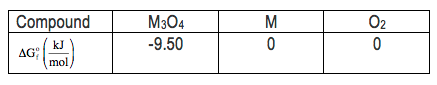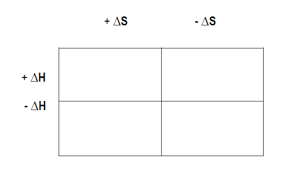Clutch Prep is now a part of Pearson
Ch.6 - Chemical EquilibriumWorksheetSee all chapters

# Chemical Thermodynamics: Gibbs Free Energy

See all sections
Sections
The Equilibrium State
The Reaction Quotient
Le Chatelier's Principle
Chemical Thermodynamics: Enthalpy
Chemical Thermodynamics: Entropy
Chemical Thermodynamics: Gibbs Free Energy
Solubilty Product Constant
Protic Acids and Bases
The pH Scale
Acid Strength

Gibbs Free Energy represents the maximum amount of work that can be done by a thermodynamic reaction at constant pressure and temperature.

###### Understanding Gibbs Free Energy

Concept #1: The signs of Gibbs Free Energy can be used to determine if a reaction is spontaneous or non-spontaneous.

Example #1: Which of the following is an example of a nonspontaneous process?

a) Ice melting at room temperature

b) Sodium metal reacting violently with water

c) Rusting of iron at room temperature

d) A ball rolling downhill

e) Water freezing at room temperature

###### Gibbs Free Energy Calculations

Example #2: Consider the decomposition of a metal oxide to its elements, where M represents a generic metal.

M3O4 (s) ⇌ 3 M (s) + 2 O(g)

i) What is the standard change in Gibbs energy for the reaction, as written, in the forward direction?

ii) What is the equilibrium constant of this reaction, as written, in the forward direction at 298 K?

iii) What is the equilibrium pressure of 0(g) over M (s) at 298 K?Example #3: For the reaction:

2 C (graphite) + H2 (g) → C2H2 (g)

ΔG° = + 209.2 kJ at 25°C. If PH2 = 100 atm, and PC2H2 = 0.10 atm, calculate ΔG for reaction.

a) +192.1 kJ
b) +266.3 kJ
c) -16.9 kJ
d) +207.8 kJ
e) +17.3 kJ

Example #4: Sodium carbonate can be made by heating sodium bicarbonate:

2 NaHCO3 (s) →  Na2CO3 (s) + CO2 (g) + H2O (g)

Given that ΔH° = 128.9 kJ/mol and ΔG° = 33.1 kJ/mol at 25°C, above what minimum temperature will the reaction become spontaneous under standard-state conditions?

a) 0.4 K

B) 3.9 K

C) 321 K

D) 401 K

E) 525 K